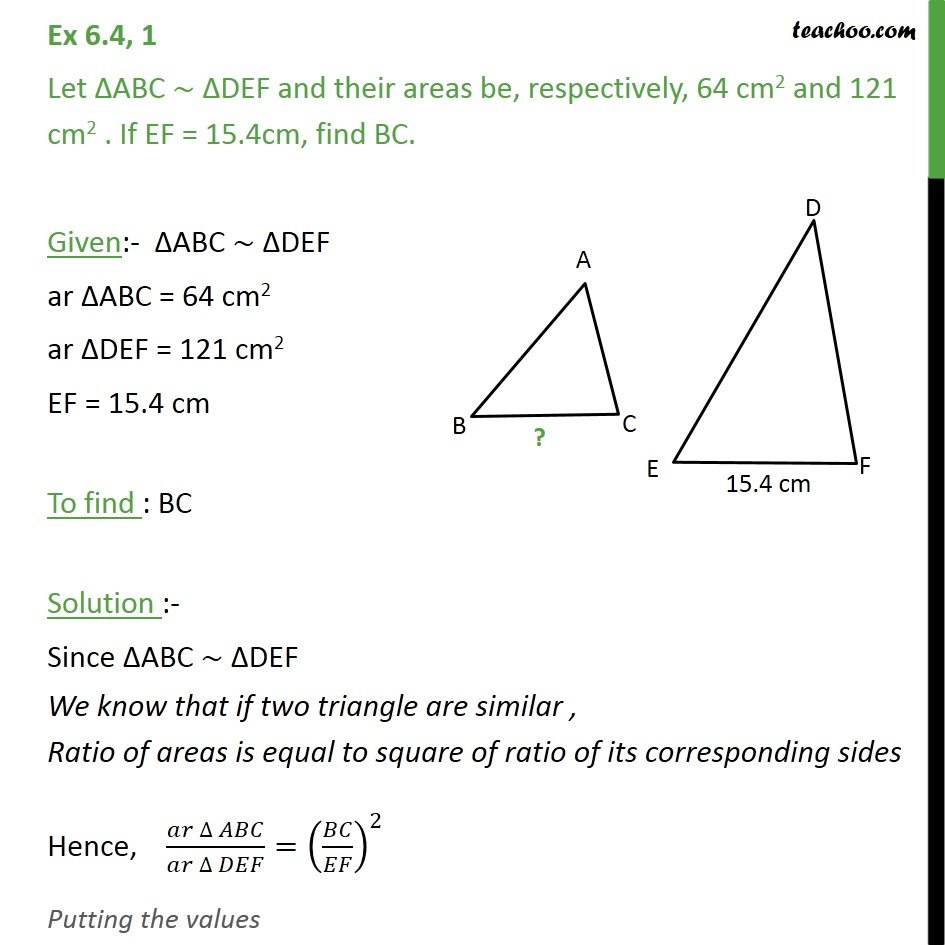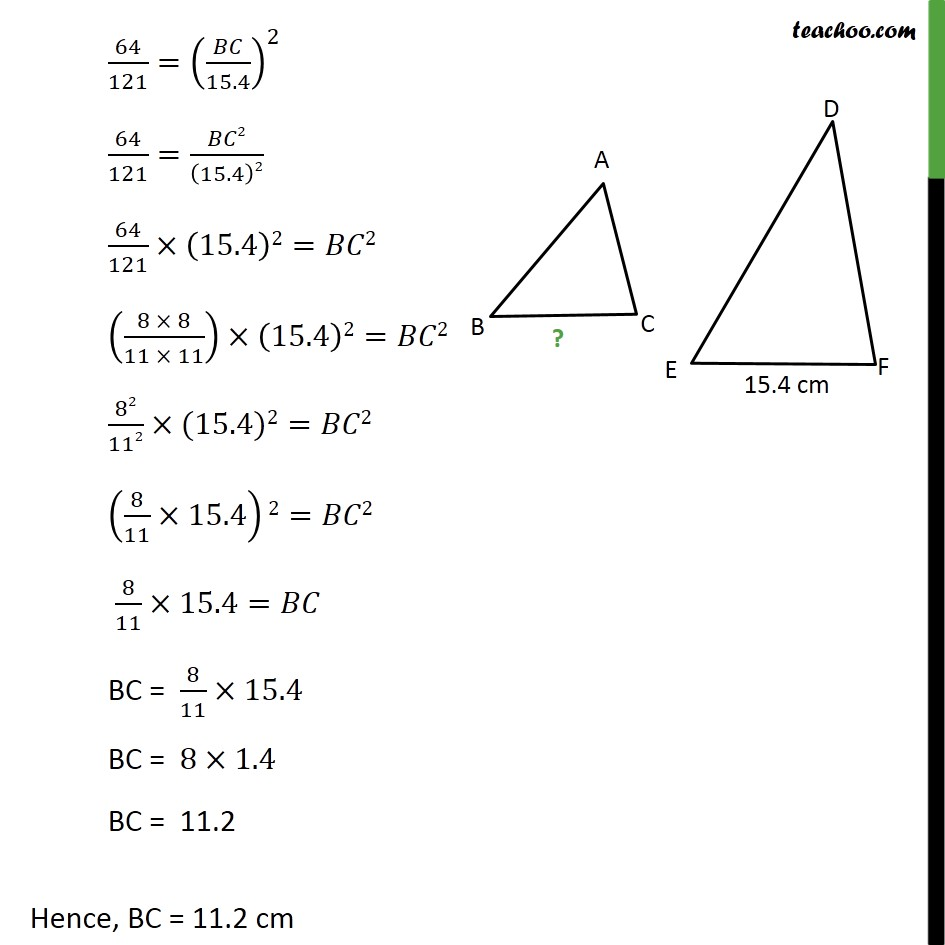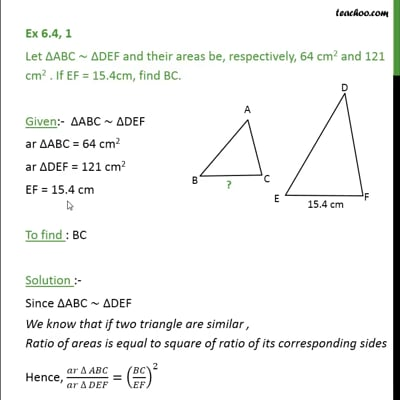Area of similar triangles

Chapter 6 Class 10 Triangles
Concept wiseThis video is only available for Teachoo black users

Solve all your doubts with Teachoo Black (new monthly pack available now!)

### Transcript

Ex 6.4, 1 Let ABC ~ DEF and their areas be, respectively, 64 cm2 and 121 cm2 . If EF = 15.4cm, find BC. Given:- ABC ~ DEF ar ABC = 64 cm2 ar DEF = 121 cm2 EF = 15.4 cm To find : BC Solution :- Since ABC ~ DEF We know that if two triangle are similar , Ratio of areas is equal to square of ratio of its corresponding sides Hence, ( )/( )=( / )^2 Putting the values 64/121=( /15.4)^2 64/121= 2/(15.4)2 64/121 (15.4)2= 2 ((8 8)/(11 11)) (15.4)2= 2 82/112 (15.4)2= 2 (8/11 15.4)2= 2 8/11 15.4= BC = 8/11 15.4 BC = 8 1.4 BC = 11.2 Hence, BC = 11.2 cm